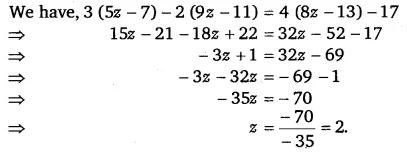# Simplify and solve the following linear equations: 3(5z – 7) – 2(9z – 11) = 4(8z – 13) – 17

Simplify and solve the following linear equations:
3(5z – 7) – 2(9z – 11) = 4(8z – 13) – 17